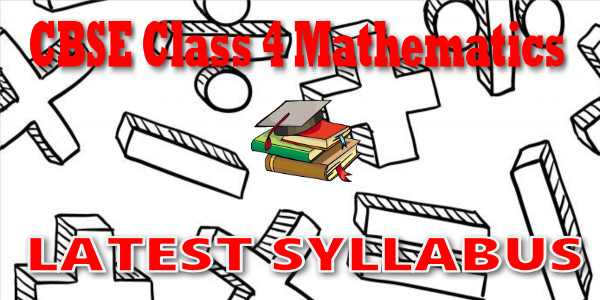# CBSE - Class 04 - Mathematics - CBSE Syllabus## CBSE, JEE, NEET, NDA

Question Bank, Mock Tests, Exam Papers

NCERT Solutions, Sample Papers, Notes, Videos

## CBSE Syllabus for Class 04 Mathematics## myCBSEguide App

Complete Guide for CBSE Students

NCERT Solutions, NCERT Exemplars, Revison Notes, Free Videos, CBSE Papers, MCQ Tests & more.

# Latest CBSE Syllabus for Class 4 MathematicsCBSE syllabus for class 4 Mathematics 2018, 2019, 2020 as per cbse.nic.in new curriculum. CBSE syllabus is available for free download in PDF format. Download latest CBSE syllabus of 4th Mathematics as PDF format. Mathematics syllabus for cbse class 4 is also available in myCBSEguide app, the best app for CBSE students.

## CBSE Academics Unit - Curriculum Syllabus

CBSE has special academics unit to design curriculum and syllabus. The syllabus for CBSE class 4 Mathematics is published by cbse.nic.in Central Board of Secondary Education, Head Office in New Delhi. The latest syllabus for  class 4 Mathematics includes list of topics and chapters in Mathematics. CBSE question papers are designed as per the syllabus prescribed for current session.

### CBSE Syllabus category

• Secondary School Curriculum (class 9 and class 10)
• Senior School Curriculum (class 11 and class 12)
• Vocational Courses (Class 11 and class 12)

CBSE Class 4
Mathematics

SHAPES & SPATIAL UNDERSTANDING

• Draws a circle free hand and with compass.
• Identifies centre, radius and diameter of a circle.
• Uses Tangrams to create different shapes.
• Tiles geometrical shapes: using one or two shapes.
• Chooses a tile among a given number of tiles that can tile a given region both intuitively and experimentally.
• Explores intuitively the area and perimeter of simple shapes.
• Makes 4-faced, 5-faced and 6-faced cubes from given nets especially designed for the same.
• Explores intuitively the reflections through inkblots, paper cutting and paper folding.
• Reads and draws 3-D objects, making use of the familiarity with the conventions used in this.
• Draws intuitively the plan, elevation and side view of simple objects.

Numbers (40 hrs.)

NUMBERS AND OPERATIONS

• Writes multiplication facts.
• Writes tables upto 10 × 10.
• Multiplies two and three digit numbers using lattice algorithm and the standard (column) algorithm.
• Divides a given number by another number in various ways such as:

– by drawing dots.

– by grouping.

– by using multiplication facts.

– by repeated subtraction.

• Applies the four operations to life situations.
• Frames word problems.
• Estimates sums, differences and products of given numbers.

MENTAL ARITHMETIC

• Adds and subtracts multiples of 10 and 100, mentally.
• Completes multiplication facts by adding partial products, mentally (e.g. 7 × 6 = 5 × 6 + 2 × 6).

FRACTIONAL NUMBERS

• Identifies half, one fourth and three- fourths of a whole.
• Identifies the symbols, 12,14,3412,14,34 .
• Explains the meaning of 12,1412,14 and 3434
• Appreciates equivalence of2424 and 12,22,33,4412,22,33,44  and 1.

Money (5 hrs.)

MONEY

• Converts Rupees to Paise.
• Adds and subtracts amounts using column addition and subtraction with regrouping.
• Uses operations to find totals, change, multiple costs and unit cost.
• Estimates roughly the totals and total cost.

Measurement (21 hrs.)

LENGTH

• Relates metre with centimetre;
• Converts metre into centimetres and vice versa.
• Solves problems involving length and distances.
• Estimates length of an object and distance between two given locations. WEIGHT
• Weighs objects using a balance and standard units.
• Determines sums and differences of weights.
• Estimates the weight of an object and verifies using a balance.

VOLUME

• Measures volumes of given liquid using containers marked with standard units.
• Determines sums and differences of volumes.
• Estimates the volume of a liquid contained in a vessel and verifies by measuring.

TIME

• Computes the number of weeks in a year.
• Correlates the number of days in a year with the number of days in each month.
• Justifies the reason for the need of a leap year.
• Reads clock time to the nearest hours and minutes.
• Expresses time, using the terms, ‘a.m.’ and ‘p.m.’
• Estimates the duration of familiar events.
• Finds approximate time elapsed by (to the nearest hour) forward counting.
• Computes the number of days between two dates.

Data Handling (6 hrs.)

• Collects data and represents in the form of bar graphs;
• Draws Inferences by discussing with the teacher.

Patterns (6 hrs.)

• Identifies patterns in multiplication and division: multiples of 9,
• Casts out nines from a given number to check if it is a multiple of nine.
• Multiplies and divides by 10s, 100s. Identifies geometrical patterns based on symmetry.## myCBSEguide

Trusted by 1 Crore+ Students

#### CBSE Test Generator

Create papers in minutes

Print with your name & Logo

3 Lakhs+ Questions

Solutions Included

Based on CBSE Blueprint

Best fit for Schools & Tutors

#### Work from Home

• Work from home with us
• Create questions or review them from home

No software required, no contract to sign. Simply apply as teacher, take eligibility test and start working with us. Required desktop or laptop with internet connection